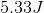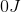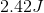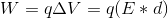# AP Physics B : Understanding Electrostatics

## Example Questions

### Example Question #1 : Ap Physics 2

Charges A and B are placed a distance of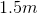from one another. The charge of particle A is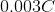whereas the charge of particle B is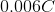. Charge B experiences an electrostatic force of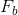from charge A. Similarly, charge A experiences an electrostatic force of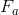from charge B.

A student places these two charges in a vacuum medium and measures. A second student places these two charges in a glass medium and measures. What is ratio of the force measured in the glass medium to the force measured in the vacuum?

The dielectric constant of glass is.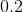Explanation:

You can simplify this question tremendously by using the definition of a dielectric constant. Dielectric constant is defined as the ratio of the electrostatic force in vacuum to the electrostatic force in the medium (in this case glass).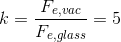The question is asking for the reciprocal of this value: the ratio of the force in glass to the force in the vacuum. Our answer is calculated by taking the reciprocal of the dielectric constant of glass.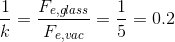### Example Question #2 : Electricity

How much work is done by the electric field moving an electron along an equipotential surface with a potential of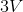?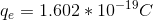We must know the strength of the electric field to solve